﻿ Common Fixed Points for Four Self-Mappings in Dislocated Metric SpacePublications are Open
Access in this journal
Article Versions
Export Article
• Normal Style
• MLA Style
• APA Style
• Chicago Style
Research Article
Open Access Peer-reviewed

### Common Fixed Points for Four Self-Mappings in Dislocated Metric Space

K. Prudhvi
American Journal of Applied Mathematics and Statistics. 2018, 6(1), 6-8. DOI: 10.12691/ajams-6-1-2
Published online: February 26, 2018

### Abstract

In this paper, we study a unique common fixed point theorem for four self mappings in dislocated metric spaces, which generalizes, extends and improves some of the recent results existing in the literature.

### 1. Introduction

In 2000, Hitzler and Seda 2 have introduced the notion of dislocated metric space in which self distance of a point need not be equal to zero and also generalized the Banach contraction principle in this dislocated metric space. Later on some of the authors like Aage, Salunke 1, sufati 3 and Shrivastava et.al., 5 have proved some fixed point theorems in dislocated metric space. In 2012, Jha and Panti 4 have proved some fixed point theorems for two pairs of weakly compatible maps in dislocated metric space. In this paper, we study a unique common fixed point theorem for four self mappings in dislocated metric space, which generalizes, extends and improves some known results existing in the references.

### 2. Preliminaries

The following definitions are due to Hitzler and Seda 2 .

Definition 2.1 2. Let X be a non-empty set and let d: X × X→ [0,∞) be a function satisfying the following conditions

(i) d(x, y) = d(y,x).

(ii) d(x, y) = d(y, x) = 0 ⇒ x = y.

(iii) d(x, y) ≤ d(x, z) + d(z, y) for all x, y, z ϵ X.

Then d is called dislocated metric or d-metric on X.

Definition 2.2 2. A sequence {xn} in a d-metric space (X, d) is called a Cauchy sequence if for given ∊ > 0 , there exists n0 ∊ℕ such that for all m, n ≥ 0, we have d(xm, xn) < ϵ.

Definition 2.3 2. A sequence {xn} in a d-metric space (X, d) converges with respect to d if there exists x ∊ X such that d(xn, x) → 0 as n→∞.

Definition 2.4 2. A d-metric space (X, d) is called complete if every Cauchy sequence is convergent with respect to d.

Definition 2.5 2. Let T and S be mappings from a metric space (X, d) itself. Then T and S are said to be weakly compatible if they commute at their coincidence point, that is, Tx = Sx for some x∊X ⇒ TSx = STx.

### 3. Main Results

Theorem 3.1. Let (X, d) be a complete d-metric space. Suppose S,T, I and J: X→X are continuous mappings satisfying :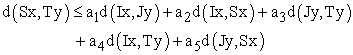(1)

for all x, y ϵ X, where ai ≥ 0 (i = 1,2,3,4,5), a1 + a2 + a3 +2a4 +2a5 < 1.

If S(X) ⊆ J(X), T(X) ⊆ I(X), and if the pairs (S, I) and (T, J) are weakly compatible then S,T, I and J have unique common fixed point.

Proof. Let x0 be an arbitrary point in X.

Since S(X)⊆ J(X), T(X)⊆I(X) there exists x1, x2 ϵ X Such that Sx0 = Jx1, Tx1 = Ix2 . Continuing this process, we define {xn} by Jx2n+1 = Sx2n , Ix2n+2 = Tx2n+1 , n = 0,1,2….. Denote y2n = Jx2n+1 = Sx2n , y2n+1 = Ix2n+2 = Tx2n+1 , n = 0,1,2…..

If y2n = y2n+1 for some n, then Jx2n+1 = Tx2n+1. Therefore, x2n+1 is a coincidence point of J and T. Also if y2n+1 = y2n+2 for some n, then Ix2n+2 = Sx2n+2. Therefore, x2n+2 is a coincidence point of I and S. Assume that If y2n ≠ y2n+1 for all n. Then we have,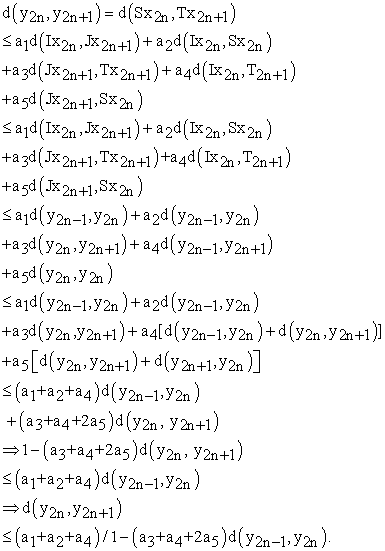Letting, b = (a1 + a2 + a4 )/1-( a3 + a4 + 2a5) < 1.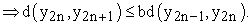This shows that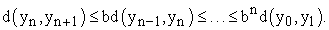For every integer m > 0, we have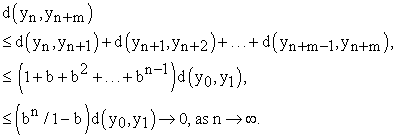Therefore, d(yn, yn+m) → 0.

⇒ {yn} is a Cauchy sequence in a complete d-metric space. So there exists a point zϵX such that yn → z. Therefore, the subsequences {Sx2n}→ z, {Jx2n+1}→ z, {Tx2n+1}→ z and {Ix2n+2}→ z. Since, T(X) ⊆ I(X), there exists a point uϵX such that z = Iu. Then we have by (1)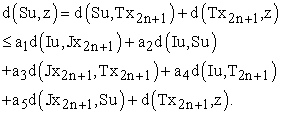Letting n→∞ we get that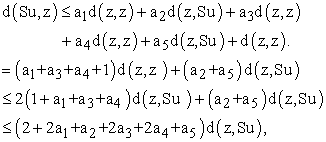which is a contradiction. So Su = z = Iu. Since, S(X)⊆J(X), there exists a point vϵX such that z = Jv.

We claim that z = Tv. If z ≠ Tv. Then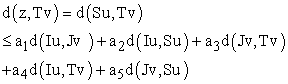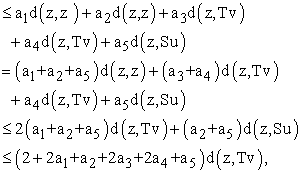which is a contradiction. So we get that z = Tv.

Therefore, Su = Iu = Tv = Jv = z. That is z is a common fixed point of S, T, f and g.

Finally in order to prove that the uniqueness of z. Suppose that z and z1, z ≠ z1 , are common fixed points of S, T, f and g respectively. Then by (1), we have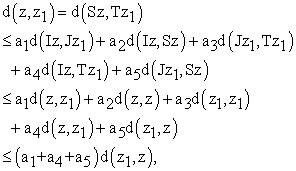which is a contradiction, since a1 + a2 + a3 +2a4 +2a5 < 1. Therefore, z = z1.

Hence, z is the unique common fixed point of S, T, f and g respectively.

Remark 3.2. If we choose f = g = I is an identity mapping in the above Theorem3.1, then we get the following corollary.

Corollary 3.3. Let (X, d) a complete d-metric space. Let S, T: X→X be continuous mappings satisfying the following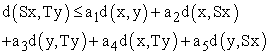for all x, yϵX, where ai ≥ 0 (i = 1,2,3,4,5), a1 + a2 + a3 +2a4 +2a5 < 1.

Then S, and T have unique common fixed point.

Remark 3.4. If we choose S = T in the above Theorem 3.1, then we get the following corollary

Corollary 3.5. Let (X, d) a complete d-metric space. Let S, T: X→X be continuous mappings satisfying the following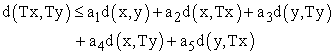for all x, yϵX, where ai ≥ 0 (i = 1,2,3,4,5), a1 + a2 + a3 +2a4 +2a5 < 1.

Then T has a unique common fixed point.

### References

  C.T. Aage and J. N. Salunke, The results on fixed point theorems in dislocated and dislocated quasi –metric space, Applied Math. Sci., 2 (59) (2008), 2941-2948. In article  P. Hitzler and A. K. Seda, Dislocated topologies, J. Electr. Engg., 51(12/s) (2000), 3-7. In article View Article  A. Isufati, Fixed point theorems in dislocated quasi metric space, Applied Mathematical Science, 4(5)(2010), 217-223. In article  K. Jha and D. Panti, A common fixed point theorem in dislocated metric space, Applied Mathematical Science, Vol.6., no. 91., (2012). 4497-4503. In article View Article  R. Shrivastava, Z.K. Ansari and M. Sharma, Some results on fixed points in dislocated and dislocated quasi-metric spaces, Journal of Advanced Studies in Topology, 3(1), (2012), 25-31. In article View Article

Published with license by Science and Education Publishing, Copyright © 2018 K. PrudhviThis work is licensed under a Creative Commons Attribution 4.0 International License. To view a copy of this license, visit http://creativecommons.org/licenses/by/4.0/